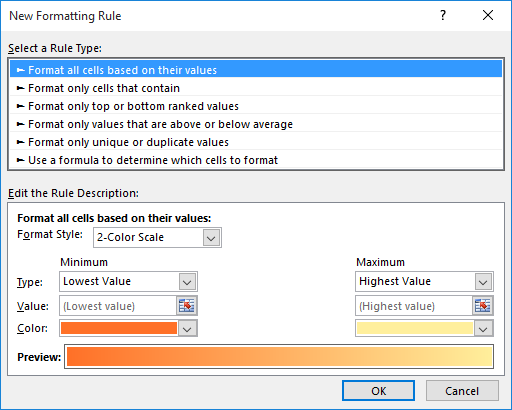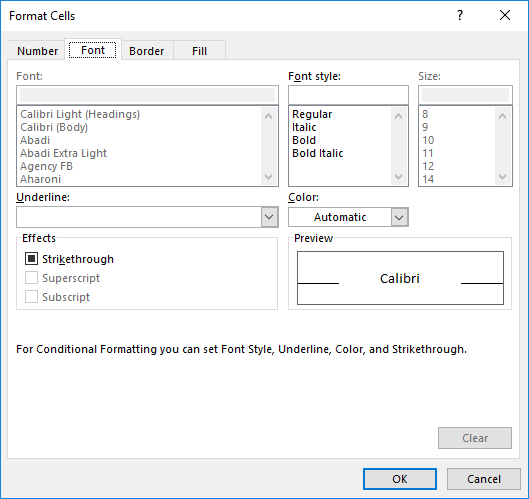# Finding Odd Values Greater Than 50Written by Allen Wyatt (last updated December 30, 2021)
This tip applies to Excel 2007, 2010, 2013, 2016, 2019, and Excel in Microsoft 365

Amol has 1,000 values in an Excel worksheet, occupying 100 rows of 10 columns each. Each value in this range is an integer value between 0 and 99. Amol needs a way to count and display all the values which are odd and greater than 50.

There are a few ways you can go about counting and displaying, but it is important to understand that these are different tasks. Perhaps the best way to display those values that fit the criteria is to use conditional formatting. You can add a conditional formatting rule to each cell that will make bold or otherwise highlight the desired values. Follow these steps:

1. Select the cells that contain your data.
2. Display the Home tab of the ribbon.
3. Click the Conditional Formatting tool in the Styles group. Excel displays a palette of options related to conditional formatting.
4. Click New Rule. Excel displays the New Formatting Rule dialog box. (See Figure 1.)
5.Figure 1. The New Formatting Rule dialog box.

6. In the Select a Rule Type area at the top of the dialog box, choose Use a Formula To Determine Which Cells to Format.
7. In the formula box enter the formula =AND(MOD(A1,2),A1>50).
8. Click the Format button. Excel displays the Format Cells dialog box. (See Figure 2.)
9.Figure 2. The Format Cells dialog box.

10. Use the controls in the dialog box to modify the formatting, as desired.
11. Click OK to close the Format Cells dialog box.
12. Click OK to close the New Formatting Rule dialog box. The formatting is applied to the range of cells you selected in step 1.

If you prefer, you could also use the following formula in step 6:

```=AND(ISODD(A1),A1>50)
```

To get the count of cells that fit the criteria, you could use an array formula:

```=SUM(MOD(MyCells,2)*(MyCells>50)
```

This formula assumes that the range of cells you want to analyze are named MyCells. Don't forget to enter the cell using Ctrl+Shift+Enter. If you don't want to use an array formula, you could use the following:

```=SUMPRODUCT((MOD(MyCells,2)*(MyCells>50))
```

You could also use a macro to derive both the cells and the count. The following is a simple version of such a macro; it places the values of the cells matching the criteria into column M and then shows a count of how many cells there were:

```Sub SpecialCount()
Dim c As Range
Dim i As Integer

i = 0
For Each c In Range("A2:J101")
If c.Value > 50 And c.Value Mod 2 Then
i = i + 1
Range("L" & i).Value = c.Value
End If
Next c

MsgBox i & " values are odd and greater than 50", vbOKOnly
End Sub
```

Note:

If you would like to know how to use the macros described on this page (or on any other page on the ExcelTips sites), I've prepared a special page that includes helpful information. Click here to open that special page in a new browser tab.

ExcelTips is your source for cost-effective Microsoft Excel training. This tip (12597) applies to Microsoft Excel 2007, 2010, 2013, 2016, 2019, and Excel in Microsoft 365.

##### Author Bio

Allen Wyatt

With more than 50 non-fiction books and numerous magazine articles to his credit, Allen Wyatt is an internationally recognized author. He is president of Sharon Parq Associates, a computer and publishing services company. ...

##### MORE FROM ALLEN

Automatically Formatting Graphics and AutoShapes

Want to change the graphics formatting defaults in Word? You can customize some of these defaults, saving yourself some time.

Discover More

Changing the Format of Existing Dates

There are a myriad of ways in which a date can be formatted�"day first, month first, number of digits in the year, etc. ...

Discover More

Displaying a Message in the Status Bar

A great place for your macro to display status information is, well, in the status bar. Displaying the information is ...

Discover MoreSave Time and Supercharge Excel! Automate virtually any routine task and save yourself hours, days, maybe even weeks. Then, learn how to make Excel do things you thought were simply impossible! Mastering advanced Excel macros has never been easier. Check out Excel 2010 VBA and Macros today!

##### More ExcelTips (ribbon)

Creating an Amortization Schedule

An amortization schedule is a report that shows how the outstanding balance on a loan changes with payments made over ...

Discover More

Averaging Based on Multiple Criteria

When you need to determine an average based on a very small selection of cells from a large dataset, based on multiple ...

Discover More

Counting Groupings Below a Threshold

When analyzing data, you may need to distill groupings from that data. This tip examines how you can use formulas and ...

Discover More
##### Subscribe

FREE SERVICE: Get tips like this every week in ExcelTips, a free productivity newsletter. Enter your address and click "Subscribe."

If you would like to add an image to your comment (not an avatar, but an image to help in making the point of your comment), include the characters [{fig}] (all 7 characters, in the sequence shown) in your comment text. You’ll be prompted to upload your image when you submit the comment. Maximum image size is 6Mpixels. Images larger than 600px wide or 1000px tall will be reduced. Up to three images may be included in a comment. All images are subject to review. Commenting privileges may be curtailed if inappropriate images are posted.

What is seven minus 1?

2021-12-30 09:46:29

Mark

Mr. Chiles,

Technically you are indeed correct. However, the original equation also works. It's using an Excel behavior that when evaluating a logical function it treats the number 0 as FALSE and all other numbers as TRUE.

A few quick checks shows this:
=AND(0, TRUE) returns FALSE
=AND(1, TRUE) returns TRUE
=AND(-1, TRUE) returns TRUE

Also, I prefer to use arithmetic operators where logicals are expected instead of AND and OR because I find them easier to read. "*" acts as AND while "+" acts as OR. I usually use them in IF statements like:

=IF( (A1 = "RED") * (A2 = "BLUE"), "A1 is RED and A2 is BLUE", "Either A1 isn't RED or A2 isn't BLUE" )
=IF( (A1 = "RED") + (A2 = "BLUE"), "A1 is RED or A2 is BLUE", "A1 isn't RED and A2 isn't BLUE" )

I suspect if Microsoft ever changes this behavior there will be a user revolt ;-)

2021-12-30 09:12:49

Rusty Chiles

Small correction to Step 6 in the conditional formatting example. The MOD portion of the formula should be appended by "=1" to account for odd values. The MOD formula divides the value in the cell by the number in the formula and the result is a remainder. Since we are looking for odd numbers, the "TRUE" solution for the first portion of AND test would be numbers whose remainder equals 1. The updated formula is =AND(MOD(A1,2)=1,A1>50)

##### This Site

Got a version of Excel that uses the ribbon interface (Excel 2007 or later)? This site is for you! If you use an earlier version of Excel, visit our ExcelTips site focusing on the menu interface.